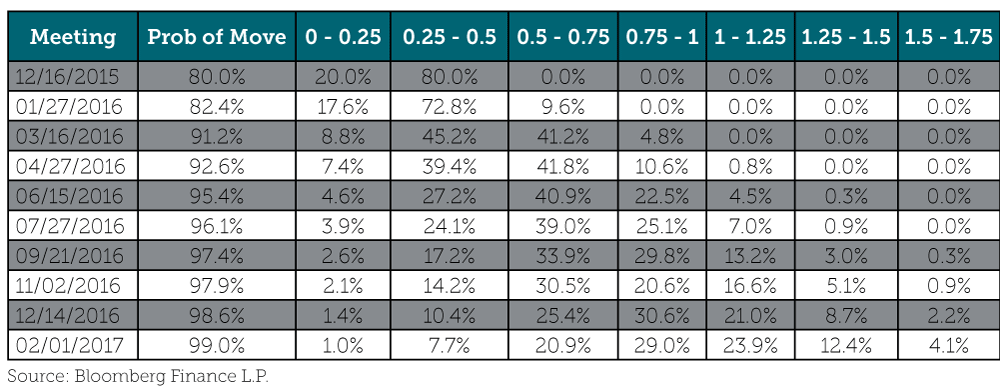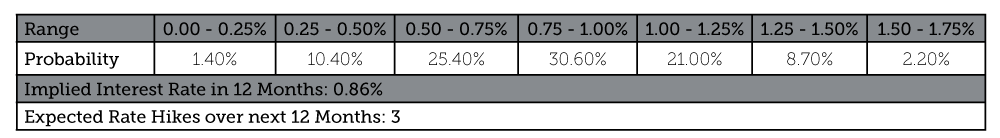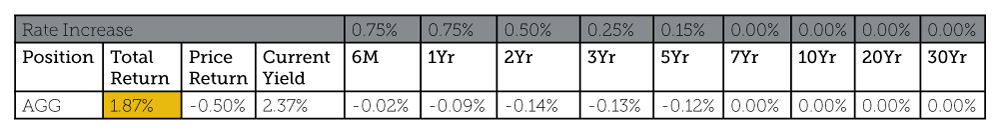Content provided by Josh Jenkins, CFA, CLS Portfolio Manager

Recent guidance from the Federal Reserve (Fed) suggests it may finally be ready to lift interest rates at its next meeting on December 16, and the market has taken note.

According to Bloomberg, as of December 10 the probability of a hike was 80%. Bloomberg has a cool function that calculates the probability of a rate hike at any given Federal Open Market Committee (FOMC) meeting based on the pricing of certain derivative contracts. This function is illustrated below and has frequently been quoted in the financial media.In addition to determining the probability of a rate move at any given meeting, the function also determines the probability of interest rates falling within a particular range. The table below illustrates this probability distribution for interest rates in December 2016. This distribution implies that the benchmark rate will be at 0.86% in 12 months. Given the benchmark rate is currently between 0.00% and 0.25% (we can assume the middle point of 0.125% for simplicity), pricing in these derivative contracts implies three rate hikes over the next 12 months.

Here’s the formula:

(Interest rate in 12 months – current interest rate) / size of each rate hike

(0.86-0.125)/0.25 = 3Three rate hikes in the next 12 months!? We should sell all our bonds right!? Wrong. Three rate increases in 12 months can be considered a slow and steady pace, and there are several reasons to believe this slow pace will not cause interest rates to rise on the long end of the curve:

• Conflicting economic and inflation data
• Nominal growth is expected to remain low
• Global interest rates are low
• Potential equity market weakness

So what could bond portfolio returns look like in this scenario (rates increase on the short end but not the long end)? Below is a simplified analysis to illustrate:The table above breaks down the components of total return for the Aggregate Bond Index (a proxy for the domestic bond market). The return components include price return and yield. From this we can see the rise in interest rates reduces the value of the bonds (price return), but the coupon payments (yield) are more than able to cover the loss. To emphasize: in this scenario there will likely be some initial volatility, but the return on fixed income can still be positive, even with a rate hike.

This simplified analysis ignores the effects of a potential increase in default rates (this would lower expected bond returns), the effects of the roll yield (this would increase expected bond returns), and convexity.